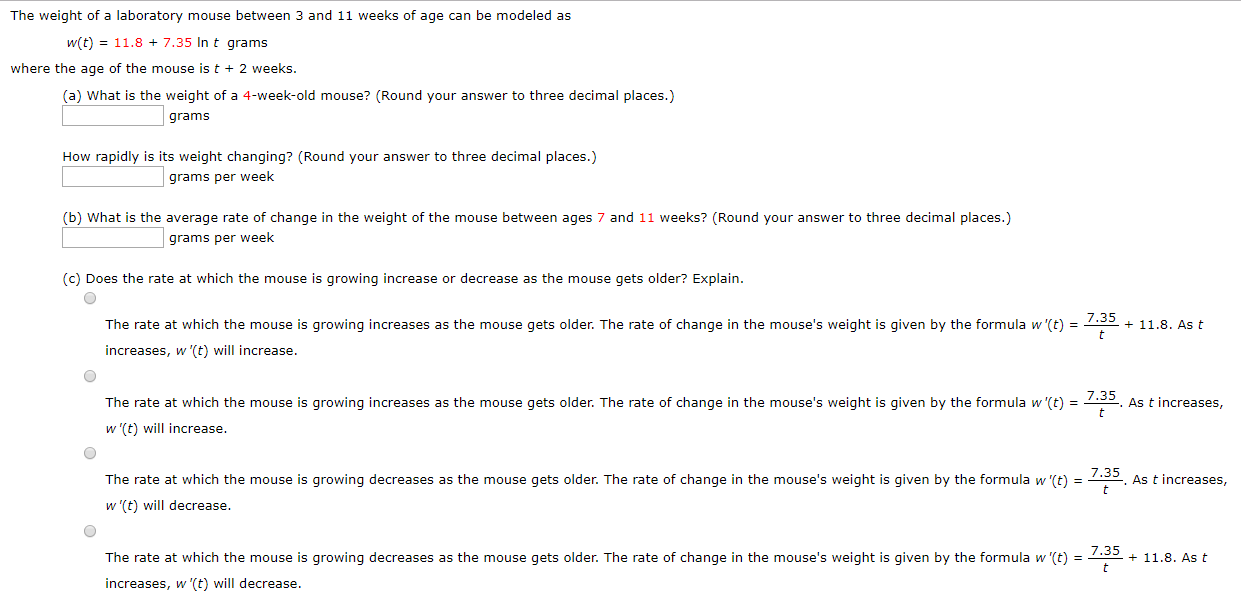# The weight of a laboratory mouse between 3 and 11 weeks of age can be modeled asw(t) 11.8 + 7.35 In t gramswhere the age of the mouse is t2 weeks.(a) What is the weight of a 4-week-old mouse? (Round your answer to three decimal places.)gramsHow rapidly is its weight changing? (Round your answer to three decimal places.)grams per week(b) What is the average rate of change in the weight of the mouse between ages 7 and 11 weeks? (Round your answer to three decimal places.)grams per week(c) Does the rate at which the mouse is qrowing increase or decrease as the mouse gets older? Explain.7.35The rate at which the mouse is growing increases as the mouse gets older. The rate of change in the mouse's weight is given by the formula w'(t)11.8. As ttincreases, w '(t) will increase.7.35As t increasestThe rate at which the mouse is growing increases as the mouse gets older. The rate of change in the mouse's weight is given by the formula w'(t)w '(t) will increase.7.35The rate at which the mouse is growing decreases as the mouse gets older. The rate of change in the mouse's weight is given by the formula w '(t) =As t increases,w (t) will decrease.7.35The rate at which the mouse is growing decreases as the mouse gets older. The rate of change in the mouse's weight is given by the formula w '(t)11.8. As tincreases, w '(t) will decrease

Question
65 viewshelp_outlineImage TranscriptioncloseThe weight of a laboratory mouse between 3 and 11 weeks of age can be modeled as w(t) 11.8 + 7.35 In t grams where the age of the mouse is t 2 weeks. (a) What is the weight of a 4-week-old mouse? (Round your answer to three decimal places.) grams How rapidly is its weight changing? (Round your answer to three decimal places.) grams per week (b) What is the average rate of change in the weight of the mouse between ages 7 and 11 weeks? (Round your answer to three decimal places.) grams per week (c) Does the rate at which the mouse is qrowing increase or decrease as the mouse gets older? Explain. 7.35 The rate at which the mouse is growing increases as the mouse gets older. The rate of change in the mouse's weight is given by the formula w'(t) 11.8. As t t increases, w '(t) will increase. 7.35 As t increases t The rate at which the mouse is growing increases as the mouse gets older. The rate of change in the mouse's weight is given by the formula w'(t) w '(t) will increase. 7.35 The rate at which the mouse is growing decreases as the mouse gets older. The rate of change in the mouse's weight is given by the formula w '(t) = As t increases, w (t) will decrease. 7.35 The rate at which the mouse is growing decreases as the mouse gets older. The rate of change in the mouse's weight is given by the formula w '(t) 11.8. As t increases, w '(t) will decrease fullscreen
check_circle

Step 1

Age of the mouse= t+2= 4 weeks

So, t=2 weeks

Step 2

Answer(a): A 4 week old mouse is 16.895 grams.

Step 3

Answer: The weight is changing at 3.67...

### Want to see the full answer?

See Solution

#### Want to see this answer and more?

Solutions are written by subject experts who are available 24/7. Questions are typically answered within 1 hour.*

See Solution
*Response times may vary by subject and question.
Tagged in

### Other